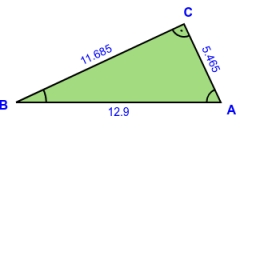# Calculate 35223

In the ABC triangle, the angles alpha, beta, and gamma are in ratio 0.4:0.2:0.9. Calculate their size.

A =  48 °
B =  24 °
C =  108 °

### Step-by-step explanation:Did you find an error or inaccuracy? Feel free to write us. Thank you!

Tips for related online calculators
Check out our ratio calculator.
Do you have a system of equations and looking for calculator system of linear equations?